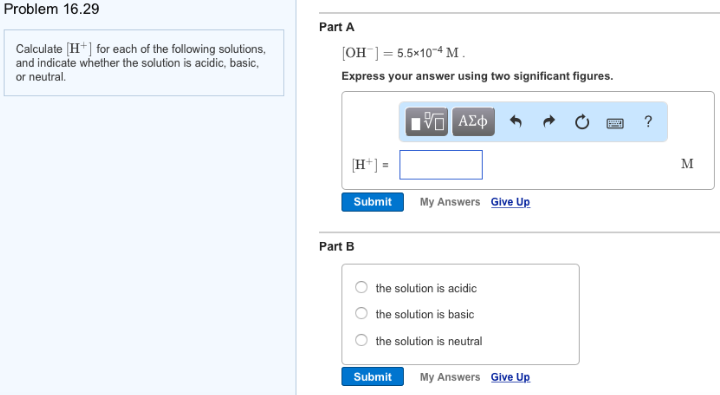# Problem: Calculate [H+] for each of the following solutions, and indicate whether the solution is acidic, basic, or neutral. Part A[OH-] = 5.5 x 10-4 M. Express your answer using two significant figures. Part B                       • the solution is acidic                       • the solution is basic                        • the solution is neutral

🤓 Based on our data, we think this question is relevant for Professor Liwosz's class at UB.

###### FREE Expert Solution###### Problem Details

Calculate [H+] for each of the following solutions, and indicate whether the solution is acidic, basic, or neutral.

Part A

[OH-] = 5.5 x 10-4 M.

Part B

• the solution is acidic

• the solution is basic

• the solution is neutral